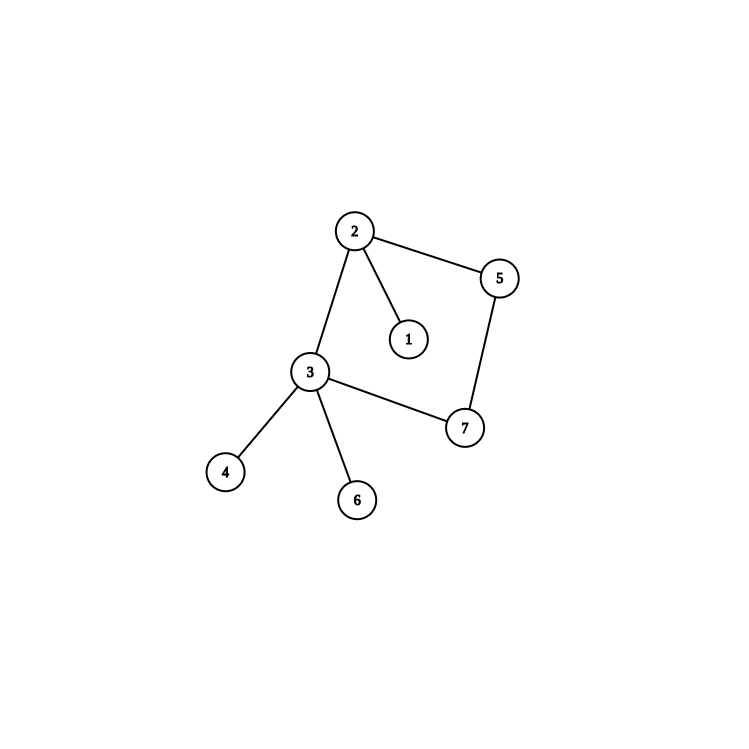# $\mathtt{Description}$

## $\mathtt{Data} \text{ } \mathtt{Range}$

$1 \le n \le 5\times10^5$

# $\mathtt{Solution}$

## $m = n$

bool flcyc;
void dfscyc(int u, int fa) {
vis[u] = true;
for (int i = head[u]; i; i = e[i].nxt) {
int v = e[i].v;
if (v != fa) {
if (vis[v]) {
flcyc = cyc[v] = cyc[u] = true;
return ;
}
dfscyc(v, u);
if (cyc[v] && flcyc) {
if (cyc[u])
flcyc = false;
cyc[u] = true;
return ;
}
}
}
}• 之前还没反悔过；
• 当前节点 $u$ 有且仅有一个没遍历过的儿子 $p$，且 $p$ 在环上；
• 要反悔到的位置（上一个还有孩子没走完的祖先的下一个要走的孩子） $q$ 满足 $q < p$

### $\mathtt{Time} \text{ } \mathtt{Complexity}$

• dfs前把所有边按照顶点排序再插入
• dfs中维护一个单调队列

ps:据说可以用一种类SA的基数排序思想使得 $\log$ 降掉。整体时间复杂度可以降为 $\mathcal{O}(n)$。不过常数较大……

# $\mathtt{Code}$

/*
* @Author: crab-in-the-northeast
* @Date: 2020-11-28 10:37:32
*/
#include <bits/stdc++.h>
int x = 0;
bool f = true;
char ch = getchar();
while (ch < '0' || ch > '9') {
if (ch == '-')
f = false;
ch = getchar();
}
while (ch >= '0' && ch <= '9') {
x = (x << 1) + (x << 3) + ch - '0';
ch = getchar();
}
if (f)
return x;
return ~(x - 1);
}
const int maxn = 500005;
const int maxm = 500005;
const int maxinf = 0x3f3f3f3f;

struct edges {
int v, nxt;
}e[maxm << 1];
int ans[maxn], cnt;
bool vis[maxn], cyc[maxn];

inline void insert(int u, int v) {
return ;
}

bool flcyc;
void dfscyc(int u, int fa) {
vis[u] = true;
for (int i = head[u]; i; i = e[i].nxt) {
int v = e[i].v;
if (v != fa) {
if (vis[v]) {
flcyc = cyc[v] = cyc[u] = true;
return ;
}
dfscyc(v, u);
if (cyc[v] && flcyc) {
if (cyc[u])
flcyc = false;
cyc[u] = true;
return ;
}
}
}
}

bool fl;
void dfs(int u, int fa, int back) {
std :: priority_queue <int, std :: vector <int>, std :: greater <int> > q;
vis[u] = true;
ans[++cnt] = u;
for (int i = head[u]; i; i = e[i].nxt) {
int v = e[i].v;
if (v != fa && !vis[v])
q.push(v);
}
while (!q.empty()) {
int v = q.top();
q.pop();
if (!fl && cyc[v] && q.empty() && back < v) {
fl = true;
return ;
}
if (!vis[v])
dfs(v, u, (!q.empty() && cyc[u]) ? q.top() : back);
}
}

int main() {
for (int i = 1; i <= m; ++i) {
insert(u, v);
insert(v, u);
}
dfscyc(1, 1);
std :: memset(vis, 0, sizeof(vis));
dfs(1, 1, maxinf);
for (int i = 1; i <= cnt; ++i)
std :: printf("%d ", ans[i]);
puts("");
return 0;
}


# $\mathtt{More}$

posted @ 2020-12-06 00:54  东北小蟹蟹  阅读(67)  评论(0编辑  收藏